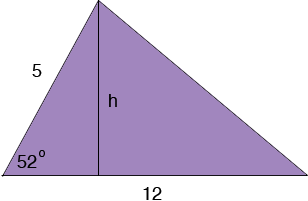SEARCH HOMEMath Central Quandaries & QueriesQuestion from Trent, a student: I need to know the area of a triangle. One side is 5 the base is 12 and the angle between them is 52 degrees. No height is specified. How do I find the height to get the area?Hi Trent,h/5 = some trig function (52o). Which trig function is it? Can you use this to find h?

PennyMath Central is supported by the University of Regina and The Pacific Institute for the Mathematical Sciences.